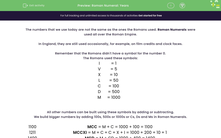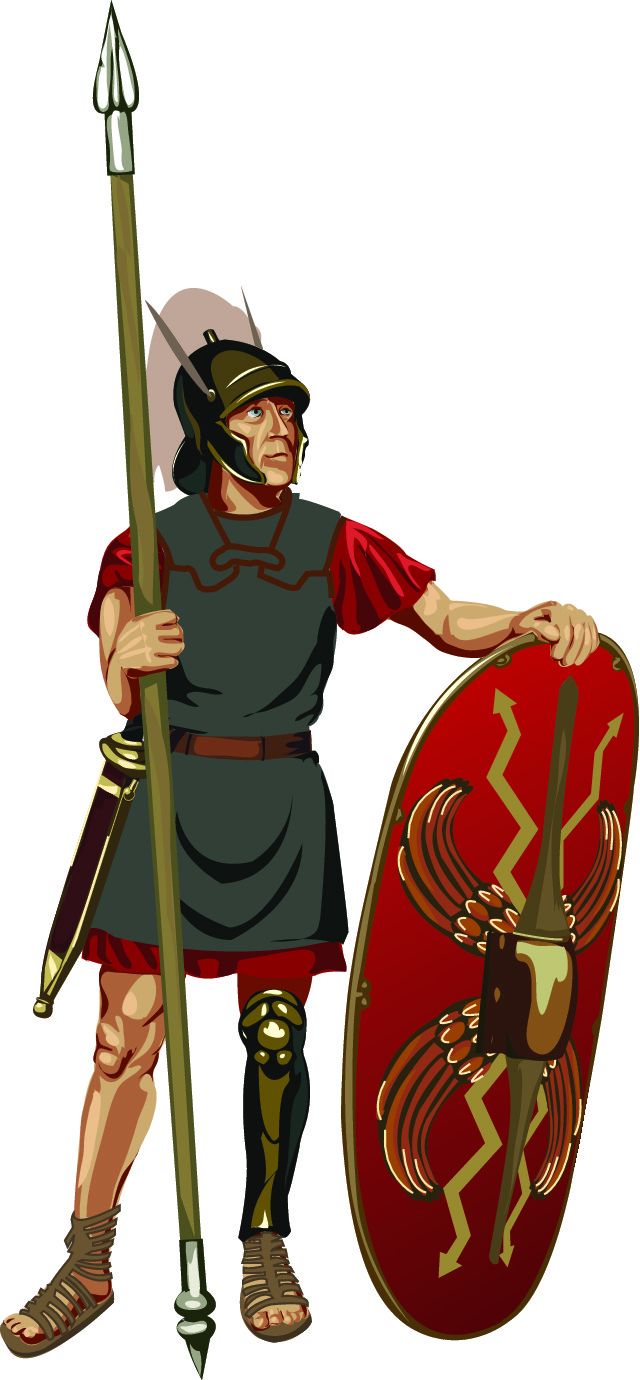# Interpret Dates Written in Roman Numerals

In this worksheet, students will convert years written in Roman numerals to normal numbers.Key stage:  KS 2

Curriculum topic:   Number: Number and Place Value

Curriculum subtopic:   Read Roman Numerals to M (1000)

Difficulty level:#### Worksheet Overview

The numbers that we use today are not the same as the ones the Romans used. Roman numerals were used all over the Roman Empire.

In England, they are still used occasionally, for example, on film credits and clock faces.

Remember that the Romans didn't have a symbol for the number 0.

The Romans used these symbols:

 I = 1 V = 5 X = 10 L = 50 C = 100 D = 500 M = 1000

All other numbers can be built using these symbols by adding or subtracting.

We build bigger numbers by adding 100s, 500s or 1000s or Cs, Ds and Ms in Roman numerals.

 MC = M + C = 1000 + 100 = 1100 MCCXI = M + C + C + X + I = 1000 + 200 + 10 + 1 = 1211 MCD = M + CD = 1000 + 400 = 1400 MCM = M + CM = 1000 + 900 = 1900

Example

Convert the years MDCCCXCV and MCMXLVIII to normal years.

Break the numbers down and then add together the bits.

MDCCCXCV = M + DCCC + XC + V = 1000 + 800 + 90 + 5 = 1895

MCMXLVIII = M + CM + XL + VIII  = 1000 + 900 + 40 + 8 = 1948

Are you ready to imagine yourself as a Roman and have a go at these dates?### What is EdPlace?

We're your National Curriculum aligned online education content provider helping each child succeed in English, maths and science from year 1 to GCSE. With an EdPlace account you’ll be able to track and measure progress, helping each child achieve their best. We build confidence and attainment by personalising each child’s learning at a level that suits them.

Get started